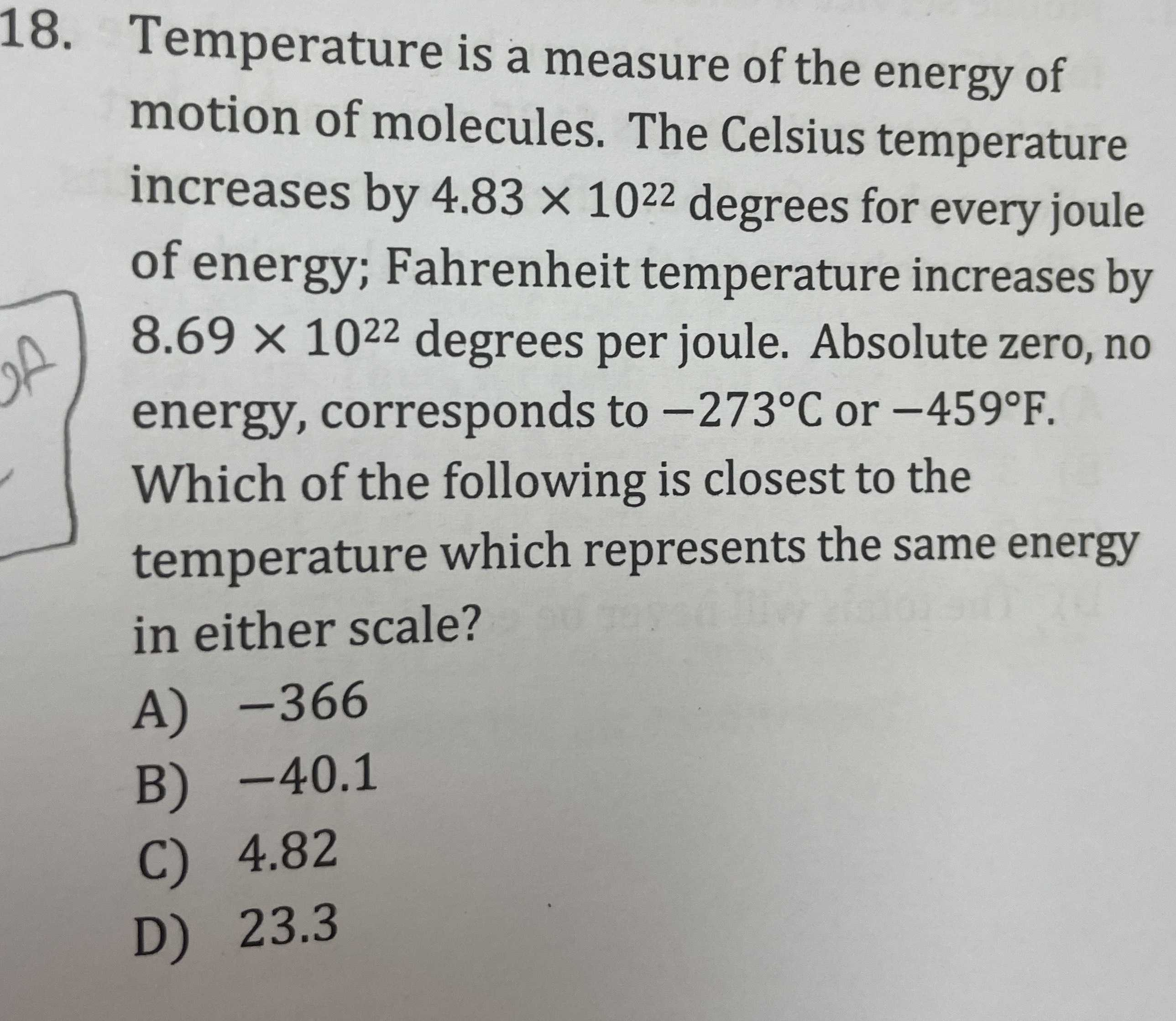### ¿Todavía tienes preguntas de matemáticas?

Pregunte a nuestros tutores expertos
Algebra
Pregunta18. Temperature is a measure of the energy of motion of molecules. The Celsius temperature increases by $$4.83 \times 10 ^ { 22 }$$ degrees for every joule of energy; Fahrenheit temperature increases by $$8.69 \times 10 ^ { 22 }$$ degrees per joule. Absolute zero, no energy, corresponds to $$- 273 ^ { \circ } C$$ or $$- 459 ^ { \circ } F$$ . Which of the following is closest to the temperature which represents the same energy in either scale?

A) $$- 366$$ B) $$- 40.1$$

C) $$4.82$$ D) $$23.3$$